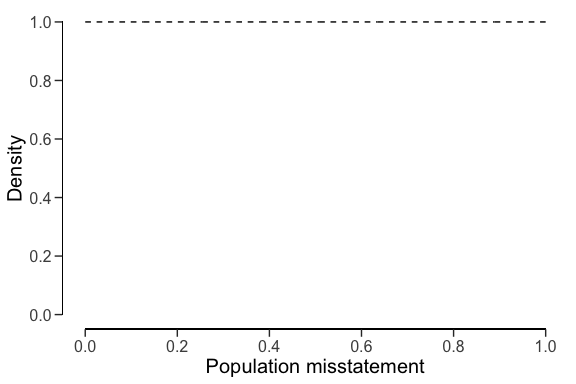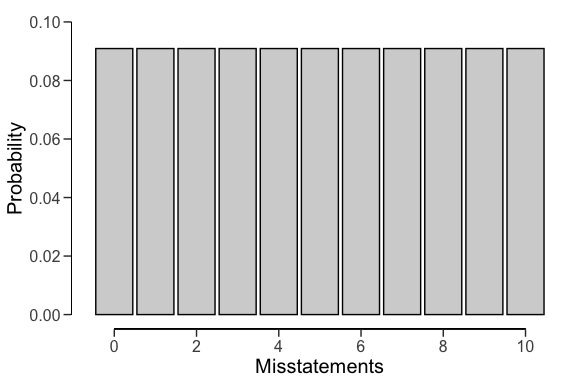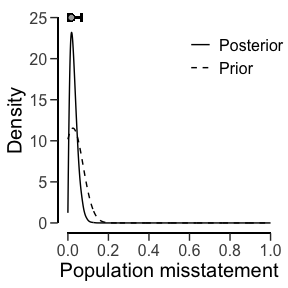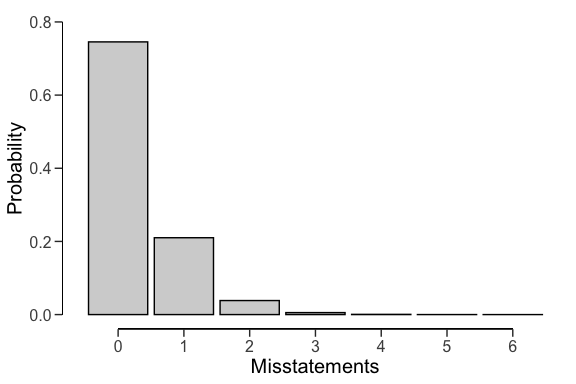# Creating a prior distribution for audit sampling

## Introduction

This vignette discusses how to set up a prior distribution for audit sampling using the auditPrior() function in the jfa package.

Bayesian statistics enables auditors to incorporate existing information about the misstatement into the sampling procedure via the prior distribution. After observing data, the information in the prior is updated to come to an overall conclusion about the misstatement in the population. The advantage of using a prior distribution is that it makes the underlying assumptions explicit and can therefore decrease the amount of audit work required to achieve the desired assurance. For example, when you have information from the auditee’s internal controls that indicates a low-risk profile, this information can be incorporated into the prior distribution in order to require less evidence from tests of details. Note that all incorporated prior information should be justified with appropriate audit evidence. What information can be incorporated into the prior distribution depends on what type of information is available, the quality of that information, and the situation at hand.

## Creating a prior distribution

The auditPrior() function is used to specify a prior distribution for audit sampling. Below is an enumeration of the several ways that a prior distribution can be constructed using this function.

### Default priors (method = 'default')

The default prior distributions are created using method = 'default'. jfa’s default priors satisfy two criteria: 1) they contain relatively little information about the population misstatement and 2) they are proper (i.e., they integrate to 1). The default priors in jfa are:

• likelihood = 'poisson': gamma($$\alpha$$ = 1, $$\beta$$ = 1)
• likelihood = 'binomial': beta($$\alpha$$ = 1, $$\beta$$ = 1)
• likelihood = 'hypergeometric': beta-binomial(N, $$\alpha$$ = 1, $$\beta$$ = 1)
• likelihood = 'normal': normal($$\mu$$ = 0, $$\sigma$$ = 1000)
• likelihood = 'uniform': uniform(min = 0, max = 1)
• likelihood = 'cauchy': Cauchy($$x_0$$ = 0, $$\gamma$$ = 1000)
• likelihood = 't': Student-t(df = 1)
• likelihood = 'chisq': chi-squared(df = 1)
• likelihood = 'exponential': exponential($$\lambda$$ = 1)
prior <- auditPrior(method = "default", likelihood = "binomial")
summary(prior)
##
##  Prior Distribution Summary
##
## Options:
##   Likelihood:                    binomial
##   Specifics:                     default prior
##
## Results:
##   Functional form:               beta(α = 1, β = 1)
##   Mode:                          NaN
##   Mean:                          0.5
##   Median:                        0.5
##   Variance:                      0.083333
##   Skewness:                      0
##   Information entropy (nat):     0
##   95 percent upper bound:        0.95
##   Precision:                     NaN

All prior distributions can be visually inspected via the plot() function.

plot(prior)Furthermore, the predict() function produces the predictions of the prior distribution on the data level for a sample of n items. For example, the command below requests the prediction of the default beta(1, 1) prior for a hypothetical sample of 6 items.

predict(prior, n = 6)
##       x=0       x=1       x=2       x=3       x=4       x=5       x=6
## 0.1428571 0.1428571 0.1428571 0.1428571 0.1428571 0.1428571 0.1428571

The predictions of the prior distribution can be visualized using the plot() function.

plot(predict(prior, n = 10))### Priors with custom parameters (method = 'param')

You can manually specify the parameters of the prior distribution with method = 'param' and the alpha and beta arguments, which correspond to the first and (optionally) second parameter of the prior as described above. For example, the commands below create a beta(2, 10) prior distribution, a normal(0.025, 0.05) prior distribution, and a Student-t(0.01) prior distribution.

auditPrior(method = "param", likelihood = "binomial", alpha = 2, beta = 10)
##
##  Prior Distribution for Audit Sampling
##
## functional form: beta(α = 2, β = 10)
## parameters obtained via method 'param'
auditPrior(method = "param", likelihood = "normal", alpha = 0.025, beta = 0.05)
##
##  Prior Distribution for Audit Sampling
##
## functional form: normal(μ = 0.025, σ = 0.05)T[0,1]
## parameters obtained via method 'param'
auditPrior(method = "param", likelihood = "t", alpha = 0.01)
##
##  Prior Distribution for Audit Sampling
##
## functional form: Student-t(df = 0.01)T[0,1]
## parameters obtained via method 'param'

### Improper priors (method = 'strict')

You can construct an improper prior distribution with classical properties using method = 'strict'. The posterior distribution of from this prior yields the same results as the classical methodology with respect to sample sizes and upper limits, but is only proper once a single non-misstated unit is present in the sample (Derks et al., 2022). For example, the command below creates an improper beta(1, 0) prior distribution.

This method requires the poisson, binomial, or hypergeometric likelihood

auditPrior(method = "strict", likelihood = "binomial")
##
##  Prior Distribution for Audit Sampling
##
## functional form: beta(α = 1, β = 0)
## parameters obtained via method 'strict'

### Impartial priors (method = 'impartial')

You can incorporate the assumption that tolerable misstatement is equally likely as intolerable misstatement (Derks et al., 2022) using method = 'impartial'. For example, the command below creates an impartial beta prior distribution for a performance materiality of 5 percent.

This method requires that you specify a value for the materiality.

This method requires the poisson, binomial, or hypergeometric likelihood

auditPrior(method = "impartial", likelihood = "binomial", materiality = 0.05)
##
##  Prior Distribution for Audit Sampling
##
## functional form: beta(α = 1, β = 13.513)
## parameters obtained via method 'impartial'

### Priors with custom prior probabilities (method = 'hyp')

You can manually assign prior probabilities to the hypothesis of tolerable misstatement and the hypotheses of intolerable misstatement (Derks et al., 2021) with method = 'hyp' in combination with p.hmin. For example, the command below incorporates the information that the hypothesis of tolerable misstatement has a probability of 60% into a beta prior distribution.

This method requires that you specify a value for the materiality.

This method requires the poisson, binomial, or hypergeometric likelihood

auditPrior(method = "hyp", likelihood = "binomial", materiality = 0.05, p.hmin = 0.6)
##
##  Prior Distribution for Audit Sampling
##
## functional form: beta(α = 1, β = 17.864)
## parameters obtained via method 'hyp'

### Priors based on the Audit Risk Model (method = 'arm')

You can translate risk assessments from the Audit Risk Model (inherent risk and internal control risk) into a prior distribution (Derks et al., 2021) using method = 'arm' in combination with the ir and cr arguments. For example, the command below incorporates the information that the inherent risk is equal to 90% and internal control risk is equal to 60% into a beta prior distribution.

This method requires the poisson, binomial, or hypergeometric likelihood

auditPrior(method = "arm", likelihood = "binomial", materiality = 0.05, ir = 0.9, cr = 0.6)
##
##  Prior Distribution for Audit Sampling
##
## functional form: beta(α = 1, β = 12)
## parameters obtained via method 'arm'

### Priors based on the Bayesian Risk Assessment Model (method = 'bram')

You can incorporate information about the mode and the upper bound of the prior distribution using method = 'bram'. For example, the code below incorporates the information that the mode of the prior distribution is 1% and the upper bound is 60% into a beta prior distribution.

This method requires the poisson, binomial, or hypergeometric likelihood

auditPrior(method = "bram", likelihood = "binomial", expected = 0.01, materiality = 0.05, ub = 0.6)
##
##  Prior Distribution for Audit Sampling
##
## functional form: beta(α = 1.023, β = 3.317)
## parameters obtained via method 'bram'

### Priors based on an earlier sample (method = 'sample')

You can incorporate information from an earlier sample into the prior distribution (Derks et al., 2021) using method = 'sample' in combination with x and n. For example, the command below incorporates the information from an earlier sample of 30 items in which 0 misstatements were found into a beta prior distribution.

This method requires the poisson, binomial, or hypergeometric likelihood

auditPrior(method = "sample", likelihood = "binomial", x = 0, n = 30)
##
##  Prior Distribution for Audit Sampling
##
## functional form: beta(α = 1, β = 30)
## parameters obtained via method 'sample'

### Priors based on a weighted earlier sample (method = 'factor')

You can incorporate information from last years results, weighted by a factor (Derks et al., 2021), into the prior distribution using method = 'factor' in combination with x and n. For example, the command below incorporates the information from a last years results (a sample of 58 items in which 0 misstatements were found), weighted by a factor 0.7, into a beta prior distribution.

This method requires the poisson, binomial, or hypergeometric likelihood

auditPrior(method = "factor", likelihood = "binomial", x = 0, n = 58, factor = 0.7)
##
##  Prior Distribution for Audit Sampling
##
## functional form: beta(α = 1, β = 40.6)
## parameters obtained via method 'factor'

### Nonparametric prior distributions (method = 'nonparam')

You can base the prior on samples of the prior distribution using method = 'nonparma' in combination with samples. For example, the command below creates a prior on 1000 samples of a beta(1, 10) distribution.

The likelihood argument is not required and will be discarded in this method

auditPrior(method = "nonparam", samples = stats::rbeta(1000, 1, 10))
##
##  Prior Distribution for Audit Sampling
##
## functional form: Nonparametric
## parameters obtained via method 'nonparam'

## Using a prior distribution

The objects returned by the auditPrior() function can be used as input for the prior argument in the planning() and evaluation() functions. Below is small demonstration of how the prior distribution can be constructed using these functions.

### Planning a sample

The prior distribution can be used the planning stage to calculate a minimum sample size by providing the objected returned by the auditPrior() function to the planning() function. For example, the command below calculates the minimum sample size to test the misstatement in the population against a performance materiality of 5%, while using a beta(1, 10) prior distribution.

prior <- auditPrior(method = "param", likelihood = "binomial", alpha = 1, beta = 10)
planning(materiality = 0.05, likelihood = "binomial", prior = prior)
##
##  Bayesian Audit Sample Planning
##
## minimum sample size = 49
## sample size obtained in 50 iterations via method 'binomial' + 'prior'

### Evaluating a sample

The prior distribution can be used the evaluation stage by providing the objected returned by the auditPrior() function to the evaluation() function. For example, the command below evaluates the misstatement in the population with respect to a performance materiality of 5% after seeing a sample of 60 items with 1 misstatement, while using a normal(0.025, 0.05) prior distribution.

prior <- auditPrior(method = "param", likelihood = "normal", alpha = 0.025, beta = 0.05)
eval <- evaluation(materiality = 0.05, x = 1, n = 60, prior = prior)
summary(eval)
##
##  Bayesian Audit Sample Evaluation Summary
##
## Options:
##   Confidence level:               0.95
##   Materiality:                    0.05
##   Hypotheses:                     H₀: Θ > 0.05 vs. H₁: Θ < 0.05
##   Method:                         poisson
##   Prior distribution:             normal(μ = 0.025, σ = 0.05)T[0,1]
##
## Data:
##   Sample size:                    60
##   Number of errors:               1
##   Sum of taints:                  1
##
## Results:
##   Posterior distribution:         Nonparametric
##   Most likely error:              0.017
##   95 percent credible interval:   [0, 0.067]
##   Precision:                      0.05
##   BF₁₀:                            4.8675
plot(eval)The predictions of the posterior distribution on the data level can be visualized using the plot() function. For example, the command below shows the predictions of the posterior distribution for a hypothetical (next) sample of 10 items.

plot(predict(eval\$posterior, n = 10))